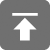# 工学1号馆

home

## HDOJ 1004 Let the Balloon Rise

Wu Yudong    August 18, 2018     HDOJ   558

Problem Description
Contest time again! How excited it is to see balloons floating around. But to tell you a
secret, the judges' favorite time is guessing the most popular problem. When the contest
is over, they will count the balloons of each color and find the result.

This year, they decide to leave this lovely job to you.

Input
Input contains multiple test cases. Each test case starts with a
number N (0 < N <= 1000) -- the total number of balloons distributed. The next N lines
contain one color each. The color of a balloon is a string of up to 15 lower-case letters.

A test case with N = 0 terminates the input and this test case is not to be processed.

Output
For each case, print the color of balloon for the most popular problem on a single line.
It is guaranteed that there is a unique solution for each test case.

Sample Input
5
green
red
blue
red
red
3
pink
orange
pink
0

Sample Output
red
pink


#include<stdio.h>
#include<string.h>
#include<math.h>
#include<ctype.h>
#include<stdbool.h>

struct color {
char s;
int n;
};

struct color Color;

char str;

void solve(void)
{
int n, i, j, k, max, sum;

while (scanf("%d", &n) && n) {
getchar();
max = sum = 0;
for (i = 0; i < n; i++) {
gets(str);
j = 0;
while (j <= i) {
if (strcmp(Color[j].s, str) == 0) {
Color[j].n++;
break;
}
j++;
}
if (i < j) {
Color[i].n = 1;
strcpy(Color[i].s, str);
sum++;
}
}
for (i = 0; i < sum; i++) {
if (max < Color[i].n) {
max = Color[i].n;
k = i;
}
}
printf("%s\n", Color[k].s);
}
}

int main(void)
{
solve();
return 0;
}